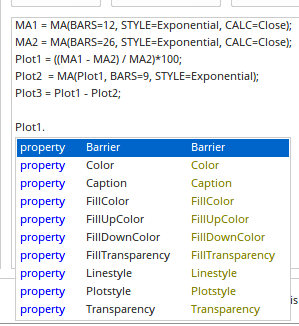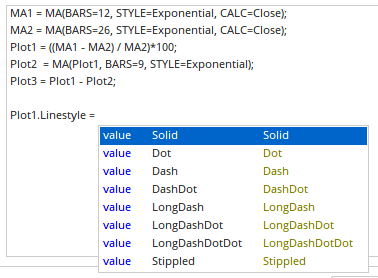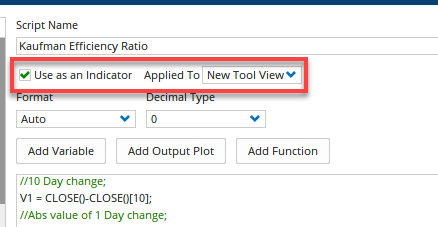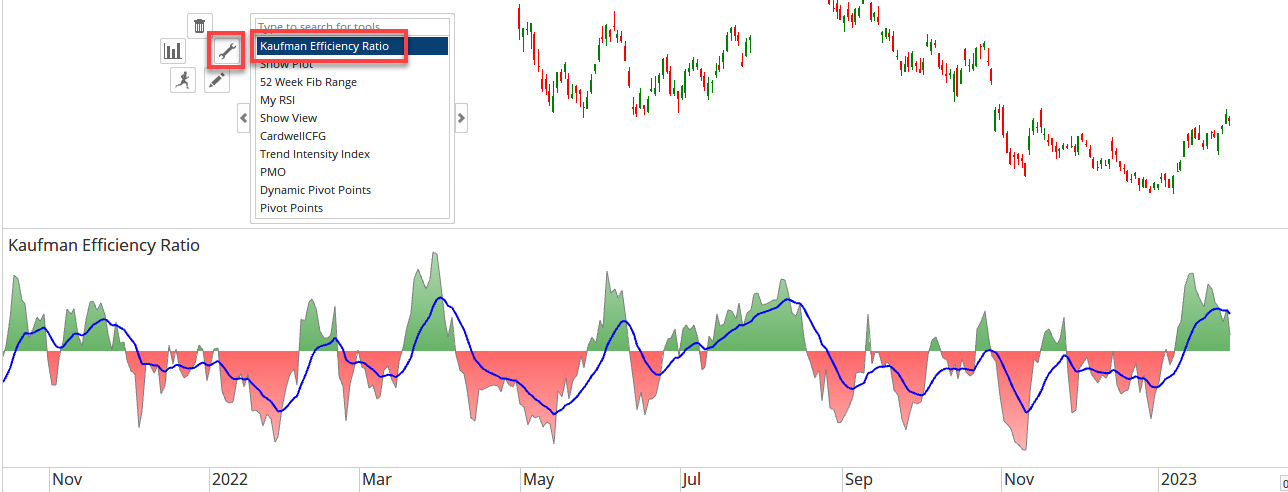# Multiple Output Plots

Author: Optuma Team Last updated: Jan 31, 2023 15:01

## Overview

Using the **Plot function it is possible to have one script formula with multiple individual outputs, with different plot styles, lines, or colours for each.

As an example, the following will calculate Kaufman’s 10 day Efficiency Ratio and also plot its 13 period EMA in the one Show View formula:

``````//10 Day chage;
V1 = CLOSE()-CLOSE();
//Abs value of 1 Day change;
V2 = ABS(CLOSE()-CLOSE());
//10 Day sum of V2;
V3 = ACC(V2, RANGE=Look Back Period, BARS=10);
//Plot ER;
Plot1=(V1/V3)*100;
Plot1.Colour = Gray;
Plot1.FillUpColour = Green;
Plot1.FillDownColour = Red;
//Plot 13EMA of ER;
Plot2=MA(Plot1, BARS=13, STYLE=Exponential, CALC=Close);
Plot2.Colour = Blue;
Plot2.LineWidth = 2;
``````

As you type Plot1. in the Script Editor, the following window will pop up allowing you to select the appropriate property, such as Line Style, Color, or Transparency:Click on the property required, and next option will appear. For example, Linestyle will allow you to choose the following:From there you can type Plot1. again on the next line and select Colour:In this example, Plot1 was shaded with green and red above and below zero, and Plot2 was a blue line for the 13EMA.

Once the complete formula has been saved as an indicator to be applied to a New View (ie in a panel below the chart), it will then be added to your toolbox and it can then be selected from the right-click menu and applied like any other tool:Available in Optuma 2.0 and later, multi plot scripts now support the Barrier colour scheme. For example:

``````O1 = OSC();<br></br>Plot1 = O1;<br></br>Plot1.Plotstyle = Histogram;<br></br>plot1.HistColScheme = Positive/Negative;<br></br>plot1.UpColour = Blue;<br></br>plot1.DownColour = Red;<br></br>plot1.Barrier = 0;<br></br>plot1.LineWidth = 2;
``````Using the above example any value lower than the set barrier (zero) shows as red and any above show as blue.